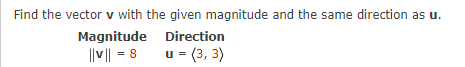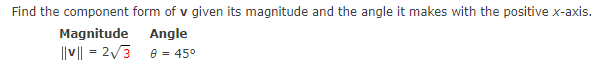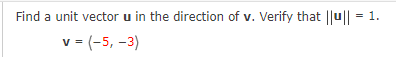Home / Expert Answers / Precalculus / nbsp-nbsp-find-the-vector-mathbf-v-with-the-given-magnitude-and-the-same-direction-a-pa626

# (Solved):     Find the vector $$\mathbf{v}$$ with the given magnitude and the same direction a ...Find the vector $$\mathbf{v}$$ with the given magnitude and the same direction as $$\mathbf{u}$$. Find the component form of $$v$$ given its magnitude and the angle it makes with the positive $$x$$-axis. Find a unit vector $$\mathbf{u}$$ in the direction of $$\mathbf{v}$$. Verify that $$\|\mathbf{u}\|=1$$. $\mathbf{v}=(-5,-3)$

We have an Answer from Expert